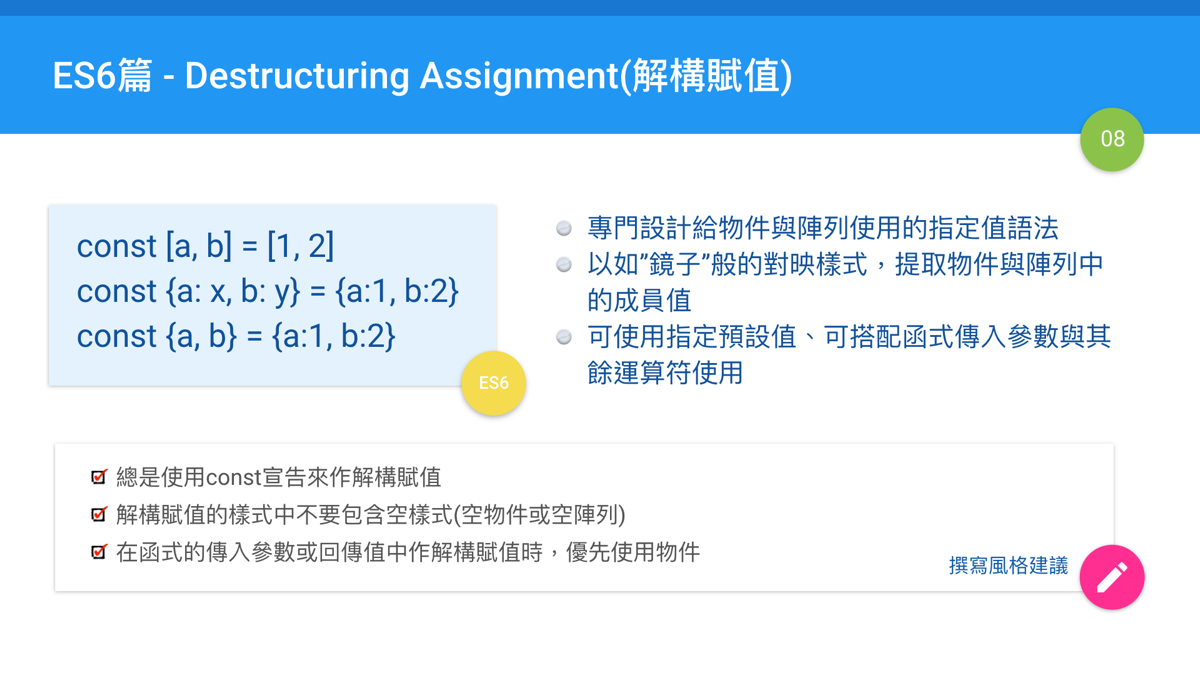#2017 iT 邦幫忙鐵人賽
DAY 8
0
Modern Web

## Day 08: ES6篇 - Destructuring Assignment(解構賦值)## 解構賦值介紹

"解構賦值"這個中文翻譯會是簡體中文的翻譯字詞，繁體中文通常會翻為"指定值"而不是"賦值"，因為網路上的中文翻譯常見的都是用這個翻譯，所以這裡用這個中文名詞。中文的意思為"解析結構來進行指定"。解構賦值是屬於ES6標準中指定運算的章節。

destructuring: 變性、破壞性。使用"解構"是對照`de-`字頭有"脫離"、"去除"的意思。

assignment: 賦值、指定。賦值通常指的是程式中使用等號(=)運算符的語句。

The destructuring assignment syntax is a JavaScript expression that makes it possible to extract data from arrays or objects using a syntax that mirrors the construction of array and object literals.

• 從陣列解構賦值
• 從物件解構賦值(或是從混用物件或陣列)
• 從非物件或非陣列解構賦值
• 解構賦值時給定預設值
• 搭配函式的傳入參數使用

## 解構賦值的各種應用情況

### 從陣列解構賦值(Array destructuring)

``````//基本用法
const [a, b] = [1, 2] //a=1, b=2

//先宣告後指定值，要用let才行
let a, b
[a, b] = [1, 2]

// 略過某些值
const [a, , b] = [1, 2, 3] // a=1, b=3

// 其餘運算
const [a, ...b] = [1, 2, 3] //a=1, b=[2,3]

// 失敗保護
const [, , , a, b] = [1, 2, 3] // a=undefined, b=undefined

// 交換值
const a = 1, b = 2;
[b, a] = [a, b] //a=2, b=1

// 多維複雜陣列
const [a, [b, [c, d]]] = [1, [2, [[[3, 4], 5], 6]]]

// 字串
const str = "hello";
const [a, b, c, d, e] = str
``````

## 從物件解構賦值(Object destructuring)

``````// 基本用法
const { user: x } = { user: 5 } // x=5

// 失敗保護(Fail-safe)
const { user: x } = { user2: 5 } //x=undefined

// 賦予新的變數名稱
const { prop: x, prop2: y } = { prop: 5, prop2: 10 } // x=5, y=10

// 屬性賦值語法
const { prop: prop, prop2: prop2 } = { prop: 5, prop2: 10 } //prop = 5, prop2=10

// 相當於上一行的簡短語法(Short-hand syntax)
const { prop, prop2 } = { prop: 5, prop2: 10 } //prop = 5, prop2=10

// ES7+的物件屬性其餘運算符
const {a, b, ...rest} = {a:1, b:2, c:3, d:4} //a=1, b=2, rest={c:3, d:4}
``````

``````// 錯誤的示範:
let a, b
{ a, b } = {a: 1, b: 2}
``````

``````let a, b
({ a, b } = {a: 1, b: 2}) //a=1, b=2
``````

``````// 混用物件與陣列
const {prop: x, prop2: [, y]} = {prop: 5, prop2: [10, 100]}

console.log(x, y) // => 5 100

// 複雜多層次的物件
const {
prop: x,
prop2: {
prop2: {
nested: [ , , b]
}
}
} = { prop: "Hello", prop2: { prop2: { nested: ["a", "b", "c"]}}}

console.log(x, b) // => Hello c
``````

## 從非陣列或非物件(原始資料類型值)解構賦值

``````const [a] = undefined
const {b} = null
//TypeError: Invalid attempt to destructure non-iterable instance
``````

``````const {a} = false
const {b} = 10
const {c} = 'hello'

console.log(a, b, c) // undefined undefined undefined
``````

``````const [a] = false
const [b] = 10
const [c] = 'hello' //c="h"

console.log( a, b, c)
``````

## 解構賦值時的預設值

`````` const [missing = true] = []
console.log(missing)
// true

const { message: msg = 'Something went wrong' } = {}
console.log(msg)
// Something went wrong

const { x = 3 } = {}
console.log(x)
// 3
``````

``````const { a ='hello' } = 'hello'
const [ b ='hello' ] = 'hello'

console.log( a, b)
``````

## 在函式傳入參數定義中使用

``````function func({a, b}) {
return a + b
}

func({a: 1, b: 2}) // 3
``````

``````function func({a = 3, b}) {
return a + b
}

func({a: 1, b: 2}) // 3
func({b: 2}) // 5
func({a: 1}) // NaN
func({}) // NaN
func() // TypeError: Cannot match against 'undefined' or 'null'
``````

``````function func({a = 3, b = 5}) {
return a + b
}

func({a: 1, b: 2}) // 3
func({a: 1}) // 6
func({b: 2}) // 5
func({}) // 8
func() // TypeError: Cannot match against 'undefined' or 'null'
``````

``````function func({a = 3, b = 5} = {}) {
return a + b
}

func({a: 1, b: 2}) // 3
func({a: 1}) // 6
func({b: 2}) // 5
func({}) // 8
func() // 8
``````

``````function func({a = 3, b = 5} = {a: 7, b: 11}) {
return a + b
}

func({a: 1, b: 2}) // 3
func({a: 1}) // 6
func({b: 2}) // 5
func({}) // 8
func() // 18
``````

``````function func({a = 1, b = 2} = {a: 10, b: 20}) {
return a + b
}

func({a: 3, b: 5}) // 8
func({a: 3}) // 5
func({b: 5}) // 6
func({a: null}) // 2
func({b: null}) // 1
func({a: undefined}) // 3
func({b: undefined}) // 3
func({}) // 3
func() // 30
``````
``````function g(a = 1, b = 2) {
return a + b
}

g(3, 5) // 8
g(3) // 5
g(5) // 7
g(undefined, 5) // 6
g(null, 5) // 5
g() // 3
``````

## React中的實例應用

### 從元件中解構出其他的物件值

``````const NavigationExampleRow = require('./NavigationExampleRow');
const React = require('react');
const ReactNative = require('react-native');

// 這是取得ReactNative其中包含內建模組的一種語法
const {
ScrollView,
StyleSheet,
} = ReactNative;

const {
``````

``````const obj = {a: 1, b: 2}
const {a: x, b: y} = obj //x=1, y=2
``````

``````const obj = {a: 1, b: 2}
const {a, b} = obj //a=1, b=2

//上面的語句相當於下面的寫法
const {a: a, b: b} = obj
``````

### 從props與state解構賦值

React的props或state裡面都有可能是巢狀的物件結構，使用解構賦值的確可以很容易的提取出裡面的屬性值，所以很常會看到類似像下面的程式碼(出自這篇文章):

``````class DataModal extends Component {
render() {
const { modalList, location: { pathname } } = this.props
const { currIndex, showModal } = this.state
// ..
}
}
``````

### 使用於函式的傳入參數之中的解構賦值

``````function DataProcess({ onClickButton, stepCurrent }) {
return (
<div>
<h2>Step {stepCurrent}</h2>
<Button onClick={onClickButton}>Click</Button>
</div>
)
}
``````

``````function DataProcess(props) {

const onClickButton = props.onClickButton
const stepCurrent = props.stepCurrent

return (
<div>
<h2>Step {stepCurrent}</h2>
<Button onClick={onClickButton}>Click</Button>
</div>
)
}
``````

## 撰寫風格建議

• 解構賦值時，優先使用const來宣告。(eslint: prefer-const)
• 解構賦值的樣式中不要包含空樣式(空物件或空陣列)。(eslint: no-empty-pattern)